Errors in the integral table of the 29th edition of
Standard Mathematical Tables and Formulae

This list contains all known corrections to the integral tables appearing in Standard Mathematical Tables and Formulae, W. H. Beyer (ed.), CRC, 29th edition, 1991, pages 217-275.

• Integral number 144 : The o '' term should be replaced with O''
• Integral number 334: For the second result, the two expressions should be separated by a plus sign, not a minus sign
• Integral number 422 : The tan x'' term should be replaced with tan ax''
• Integral number 607 : Needs the constraints: n>0, m>0
• Integral number 611 : The constraint p<1'' should be 0<p<1''
• Integral number 612 : Is incorrect
• Integral number 618 : Needs the constraint: a>0
• Integral number 619 : Needs the constraints: a>0, m>-1, n>-2
• Integral number 629 : Needs the constraint: m is an integer
• Integral number 630 : The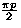'' term should be replaced with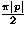''
• Integral number 633 : The'' term should be replaced with''
• Integral number 643 : The constraint  a<1'' should be |a|<1''
• Integral number 646 : The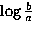'' term should be replaced with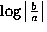''
• Integral number 647 : The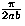'' term should be replaced with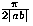''
• Integral number 653 : Needs the constraint: m=0,1,2,3,...
• Integral number 663a: Needs the constraint: a>0
• Integral number 663b: Needs the constraint: a>0
• Integral number 666 : Needs the constraints: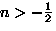,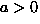• Integral number 667 : Needs the constraint: n>-1
• Integral number 669 : The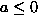'' term should be replaced with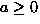''
• Integral number 670 : Needs the constraint: n>0
• Integral number 671 : Needs the constraint: n>0
• Integral number 677 : Needs the constraint: n>-1
• Integral number 679 : The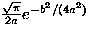'' term should be replaced with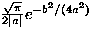''
• Integral number 684 : Needs the constraint: n>-1
• Integral number 712 : The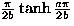'' term should be replaced with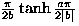''
• Integral number 712 : The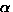'' term should be replaced with a ''
• Integral number 713 : The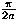'' term should be replaced with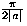''
• Integral number 714 : Needs the constraint: a>0
• Integral number 717 : Needs the constraint: b>0
• Integral number 718 : Needs the constraint: b>0

Errata for integral tables in 29th edition of STANDARD TABLES and FORMULAE